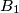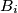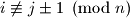### IMO Shortlist 2008 problem C1

Kvaliteta:
Avg: 0,0
Težina:
Avg: 6,0
In the plane we consider rectangles whose sides are parallel to the coordinate axes and have positive length. Such a rectangle will be called a box. Two boxes intersect if they have a common point in their interior or on their boundary. Find the largest$n$ for which there exist$n$ boxes$B_1$,$\ldots$,$B_n$ such that$B_i$ and$B_j$ intersect if and only if$i\not\equiv j\pm 1\pmod n$.

Proposed by Gerhard Woeginger, Netherlands
Izvor: Međunarodna matematička olimpijada, shortlist 2008